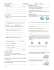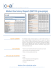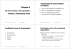# Review Questions

## Transcription

Review Questions
```Chapter 20 Review Questions
Name__________________
20.1 I can define the basics of electrostatics, conservation and charge interactions
1. If an object is attracted by a positively charged rod, we can be sure that the body is:
a) negatively charged
b) losing electrons
c) either neutral or negative
2. As a positively charged object is brought steadily nearer to (but not touching) the knob of a
positively charged electroscope, the leaves of the electroscope:
a) are not effected
c) come closer together
3. Like charges repel, whereas opposite charges attract.
a) True
b) False
4. Electric charge can be created and destroyed, but the charges must be created and destroyed
in pairs (one + and one - )
a) True
b) False
5.
a.
b.
c.
d.
Electric forces can be either repulsive or attractive, whereas gravitational force is always:
Attractive
Repulsive
both a and b
neither a nor b
6.
a.
b.
c.
Metals contain _____ electrons; rubber has _____ electrons.
bound, free
free, bound
excess, insufficient
7.
a)
b)
c)
d)
Charge carriers in a metal are electrons rather than protons, because electrons are
relatively far from a nucleus.
loosely bound.
Both a and b
none of the above
20.2 Describe three methods of charging (friction, conduction, and induction)
8. A plastic ruler is rubbed with a towel and becomes charged. The towel is brought near an
electroscope (like my pop can electroscope) and it is found to be charged. If I then bring my
ruler to the electroscope, it should be:
a) Neutral (not charged)
b) oppositely charged as the towel
c) the same charge as the towel
9. When a person standing on the ground touches a rod that is positively charged, the person:
a) gives the rod extra electrons
b) gives the rod extra protons
c) accepts the rods extra protons
d) accepts the rods extra electrons
10. Objects can be charged by
a) touching (conduction)
b) induction.
c) friction.
d) all of the above
e) none of the above
11. Bring a charged object near a conductor and then momentarily ground it by touching one part.
This demonstrates charge by
a) Induction.
b) electrification.
c) polarization.
d) deduction.
12. Pie tins fly off the Van de Graaff generator because the generator charges them and then repel
each other.
a. True
b. False
13. Charged objects are attracted to neutral objects because of polarization
a. True
b. False
20.3 Draw the redistribution of electric charges on a neutral object when a charged object is
brought near and explain why an attractive force results from bringing a charged object near a
neutral object.
14. A negatively charged balloon sticks to a wooden door. However, an uncharged balloon does
not stick to a wooden door. What is the nature of the charge on the wooden door?
a) Electrically neutral
b) Positively charged
c) Negatively charged
d) Cannot be determined
20.4 Calculate the electric force between charged objects
15. What is the force between two small charged spheres that have charges of 2.0x10–7 C and
3 10–7 C and are placed 30 cm apart in air?
a) 6 x 10-7 N
b) 0.006 N
c) 0.6 N
16. A charge of +6mC is 40cm from a charge of +8mC. What is the magnitude of the force
between them?
a. 2.7x106 N
b. 2.7x108 N
c. 1.4x106 N
d. 1.4x108 N
20.5 Determine the new electric force on charged objects after they touch and are then
separated.
17. One metal sphere has a charge of +10C and another has a charge of +8C. They are allowed
to touch and are separated to a distance of one meter. What is the force between them?
a) 7.29 x 1011 N
b) 7.29 N
c) 7.29 x 106N
Answer: A (When they touch, the spheres will share charge equally, + 9C each. Calculate the force.
20.6 Predict electrostatic force and strength of electric field based on relationship between
variables
18. Particle A has twice as much charge as particle B. Compared to the force on particle A, the
force on particle B is
a) two times as much.
b) four times as much.
c) the same.
d) half as much.
e) none of the above
19. _____ vary/varies inversely as the square of the distance between two point objects.
a. Gravitational force
b. Electric force
c. Neither a nor b
d. Both a and b
20. If the distance between two charges becomes 1/6 as much, what is the new force between
them?
a. 1/36 N
b. 36 times as much as the original force
c. 6 N
d. 6 times the original force
=========================================================================
1. Electrons carry a
a. positive charge.
b. Negative charge
c. neutral charge.
d. variable charge.
Diff: 1
3) Since there is a universal Law of Conservation of Charge
A) charges can be annihilated but not created.
B) charges cannot be created or destroyed.
C) charges can be created and destroyed.
Diff:2
4) Which is true?
A) Unlike charges repel.
B) All charges attract.
C) Unlike charges attract.
D) Like charges attract.
Diff: 1
5) A neutral atom has
A) equal numbers of electrons, protons, and neutrons.
B) equal numbers of protons and neutrons.
C) equal numbers of electrons and neutrons.
D) equal numbers of electrons and protons.
E) zero neutrons.
Dijf: 1
7) Charge is
A) invariant.
B) conserved
C) quantized.
D) invariant, conserved, and quantized
Diff:l
10) The two types of charge (plus and minus) were named by
A) Lord Rutherford.
B) Benjamin Franklin.
C) Albert Einstein.
D) Richard Feynman.
E) Newton.
Diff:l
11) Can you get an electric shock by touching a charged insulator?
A) only if you are insulated
B) yes
C) no
Diff:l
1) A glass rod is rubbed with a piece of silk. During the process the glass rod acquires a positive
charge and the silk
A) acquires a positive charge also.
B) remains neutral.
C) acquires a negative charge.
D) could either be positively charged or negatively charged. It depends on how hard the rod
was rubbed.
Diff:1
2) Explain how objects can get charged by FRICTION.
Answer: When two dissimilar materials are rubbed together, one literally rubs or tears electrons off
the one type of molecule, leaving it positively charged, and the other material acquires the extra
electrons, thereby becoming negative.
Diff: 2
3) The charge carried by one electron is e = -1.6 x 10-19 C. The number of excess electrons
necessary to produce a charge of 1.0 C is
A) 6.3 x 1018.
B) 1.6 x 1019.
C) 6.3 x 109.
D) 6.3 x 106.
E) 1.6 x 1018.
Diff:2
4) When an electron is removed from a neutral atom, it becomes
A) a positive ion.
B) a negative ion.
C) heavier.
D) a bipolar atom.
E) more massive.
Diff:l
Electric Force
Choose from the following:
(a) C2/N-m2
(b) N-m2/C2
(c) coulomb
(d) N/C
(e) dimensionless
(f) j/C2
1) The Coulomb constant has which of the following units?
Diff 1
4) Consider a container of 2.0 grams of hydrogen gas (one gram mole). Suppose you removed all the
electrons and moved them to the other side of the Earth (Earth diameter 12740. km). (12.04 x 1023
electrons)
(a) How much charge is left behind?
(b) What is the attractive force between the protons here and the electrons at the other side of
the Earth?
Answer: (a) 1.9 x 105 Coulombs
(b) 2.1 x 106 newtons = 2.1 x 102 tons!
Diff3
6) Ben Franklin named charges "plus" and "minus". Suppose he had gotten it reversed and had
called plus charges "minus" and called minus "plus", what would have been the consequences?
Answer: Like charges would still repel, unlike charges would attract. Coulomb's Law would still
describe the electric force.
Diff: 3
9) Only gravitation determines the planetary orbits. Why aren't the other fundamental forces (and
electromagnetism in particular) important for the motions?
Answer: Only gravity and electromagnetism are long range, however the planets and satellites are
electrically neutral. The planets do not have "negative mass" to neutralize the positive mass so
gravity predominates.
Diff: 2
10) The force which binds, or holds, atoms together to form molecules is
A) gravitational.
B) magnetic.
C) friction.
D) nuclear.
E) electrical.
Diff: 1
11) How far apart should two protons be if the electrical force of repulsion is equal to the weight
(attraction to the Earth) of one of the protons?
Diff: 2
12) A charged rod will attract a thin stream of water falling from a faucet even though the water is
neutral, it has no net charge. How can the rod attract the water?
Answer: The Coulomb force of the rod polarizes each molecule of water (one sign of charge is
attracted and the opposite sign is repelled). The charge that is attracted feels a slightly larger
attraction than the opposite charge is repelled because it is at a slightly different distance from the
rod.
Diff:2
13) Two charged objects attract each other with a force F. What happens to the force between them if
one charge is doubled, the other charge is tripled, and the separation distance between their centers
is reduced to one-fourth its original value? The force is now equal to
A) 24 F.
B) 6/16 F.
C) 16F.
D) 96 F.
E) 8/22 F.
Diff:2
15) Two charged objects attract each other with a certain force. If the charges on both objects are
doubled with no change in separation, the force between them
A) becomes zero.
B) doubles.
C) halves.
E) increases, but we can't say how much without knowing the distance between them.
Diff:2
18) Consider point charges of +Q and +4Q which are separated by 3 m. At what point, on a line
between the two charges, would it be possible to place a charge of -Q such that the electrostatic force
acting on it would be zero?
A) 3/5 m from the +Q charge
B) 1m from the +Q charge
C) 1m from the +4Q charge
D) 3 m from the +4Q charge
E) There is no such point possible.
Oiff: 2
20) Two charges are separated by 5.0 meters and attract one another. If one charge is halved, the
other tripled, and the distance quartered, by what factor does the attraction change?
A) 48
B) 3
C) 2
D) 24
E) 16
Oiff 2
22) Sphere A carries a net charge and sphere B is neutral. They are placed near each other on an
insulated table. Which statement best describes the electrostatic force between them?
A) There is no force between them since one is neutral.
B) There is a force of repulsion between them.
C) There is a force of attraction between them.
D) The force is attractive if A is charged positively and repulsive if A is charged negatively.
Diff 2
23) Charge +2q is placed at the origin and charge -q is placed at x = 2a. Where can a third positive
charge +q be placed so that the force on it is zero?
Diff: 3
Choose from the following:
(a) C2/N-m2
(d) N/C
(b) N-m2/C2
(e) dimensionless
(c) coulomb
(f) J /C2
25) Charge has which of the units shown above?
Diff: 1
26) An electron and a proton are separated by a distance of 1 m. What happens to the size of the
force on the proton if the electron is moved 0.5 m closer to the proton?
A) It increases to 4 times its original value.
B) It decreases to one-half its original value.
C) It increases to 2 times its original value.
D) It decreases to one-fourth its original value.
E) It increases to 8 times its original value.
Diff:2
16) One kilogram is hung from a string and it stretches 4.0 cm. A charge of 3.0 µC is placed on the
kilogram. How much charge must be placed 4.0 cm below the kg (before it moves) so that the kg
moves up by 1.0 cm to a new equilibrium position?
Diff: 2
19) A point charge of +Q is placed at the center of a square, and a second point charge of -Q is
placed at the upper-left comer. It is observed that an electrostatic force of 2 N acts on the positive
charge at the center.
What is the magnitude of the force that acts on the center charge if a third charge of -Q is placed at
the lower-left comer?
A) zero
B) √ N
C) 4 N
D) √
Oiff: 2
21) Two volleyballs, each of mass 0.300 kg, are charged by an electrostatic generator. Each is
attached to an identical string and suspended from the same point. They repel each other and hang
with separation 50.0cm. The length of the string from the point of support to the center of a ball is
250. cm.
Determine the charge on each ball.
Diff: 2
3) Consider an equilateral triangle with charges 69. µc,24 µc,and 24 µC at each respective vertex.
Each charge is 31.cm from its neighbors.
(a) What is the magnitude of the total electrostatic force on the 69. µC charge?
(b) If the charges were 3.5 times farther apart, what would be the force on the 69. µC charge?
(b) 3.9 N
Diff3
2) Consider the x-axis with 4.0 µC at x = 1.0 meter and -2.0 µC at the origin. Where should 6.0 µC be
placed so that the net force on it is zero?
Oiff: 2
5) There is a 5.0 µC charge at each of 3 corners of a square (each side 70. mm long). What is the
force on +6.0 µC placed at the center of the square?
Answer: 28. Newtons toward the empty corner
Oiff: 2
14) A point charge of +Q is placed at the centroid of an equilateral triangle. When a second charge of
+Q is placed at one of the triangle's vertices, an electrostatic force of 4 N acts on it.
What is the magnitude of the force that acts on the center charge when a third charge of +Q is placed
at one of the other vertices?
A) zero
B) 4 N
C) 8 N
D) 16 N
Diff: 3
```

#### Similar documents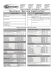### Ex1_Review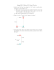### MAGNETISM, n. Something acting upon a magnet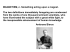### Note, Personal Checking Account is selected Note, Woodmans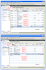### Review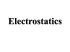### Physics Worksheet Lesson 16 Electrostatics